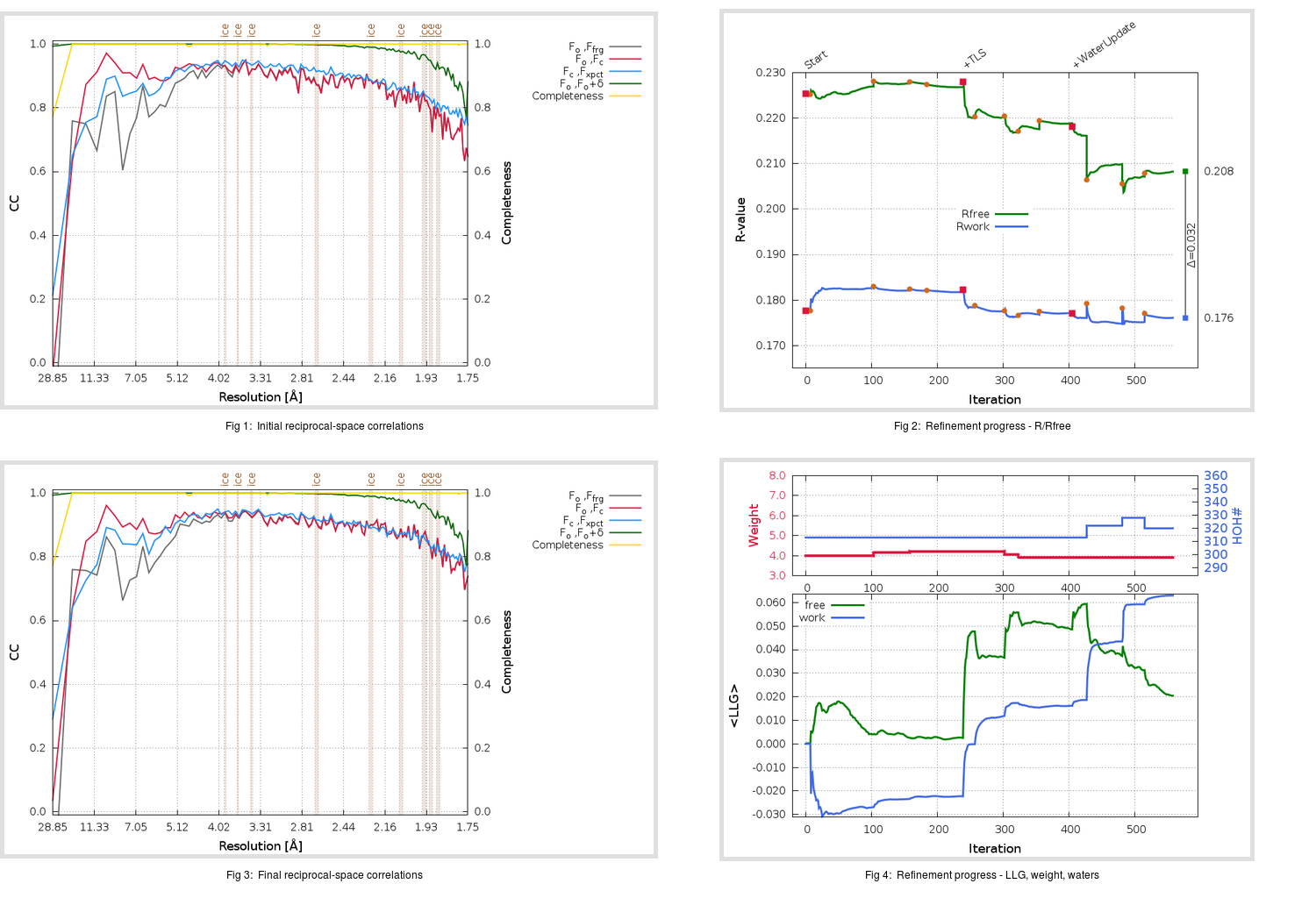Content:

```    Diffraction limits & principal axes of ellipsoid fitted to diffraction cut-off surface:
1.729         0.9288   0.0000   0.3706       0.997 a* + 0.076 c*
1.699         0.0000   1.0000   0.0000       b*
1.683        -0.3706   0.0000   0.9288      -0.696 a* + 0.719 c*
```

## Deposited

` `
 Date deposited Date data collection Resolution R, Rfree 20200215 20200201 1.75 0.1712 0.2224

Molprobity (CCP4 7.0 version) summary:

```Ramachandran outliers =   0.33 %
favored =  98.03 %
Rotamer outliers      =   3.79 %
C-beta deviations     =     0
Clashscore            =   2.34
RMS(bonds)            =   0.0142
RMS(angles)           =   1.83
MolProbity score      =   1.45
Resolution            =   1.75
R-work                =   0.1712
R-free                =   0.2224
```

```Number of waters      =   313

<B> (all atoms) =   27.92 ( sd =   10.78 ) for       2685 non-hydrogen atoms
<B>   (protein) =   26.71 ( sd =   10.26 ) for       2372 non-hydrogen atoms
<B>     (water) =   37.07 ( sd =   10.29 ) for        313 non-hydrogen atoms
<B>    (others) =    0.00 ( sd =    0.00 ) for          0 non-hydrogen atoms

B min/max       (all non-hydrogen atoms) =   13.12 /  127.06
B min/max   (protein non-hydrogen atoms) =   13.12 /   80.42
B min/max     (water non-hydrogen atoms) =   15.47 /  127.06
B min/max     (other non-hydrogen atoms) =    0.00 /    0.00
```

## BUSTER (re-)refinement

` `

Molprobity (CCP4 7.0 version) summary:

```Ramachandran outliers =   0.00 %
favored =  97.70 %
Rotamer outliers      =   3.79 %
C-beta deviations     =     0
Clashscore            =   1.28
RMS(bonds)            =   0.0114
RMS(angles)           =   1.54
MolProbity score      =   1.36
Resolution            =   1.75
R-work                =   0.1761
R-free                =   0.2083
```

```Number of waters      =   320

<B> (all atoms) =   28.80 ( sd =    9.68 ) for       2692 non-hydrogen atoms
<B>   (protein) =   27.32 ( sd =    8.59 ) for       2372 non-hydrogen atoms
<B>     (water) =   39.77 ( sd =   10.26 ) for        320 non-hydrogen atoms
<B>    (others) =    0.00 ( sd =    0.00 ) for          0 non-hydrogen atoms

B min/max       (all non-hydrogen atoms) =   14.17 /   89.44
B min/max   (protein non-hydrogen atoms) =   14.17 /   69.25
B min/max     (water non-hydrogen atoms) =   16.28 /   89.44
B min/max     (other non-hydrogen atoms) =    0.00 /    0.00
```

Refinement progression:Results:

` `
 File Remark 6Y2E_aB_refine.01_03_refine.pdb.gz exact refinement commands are in header 6Y2E_aB_refine.01_03_refine.mtz.gz including original deposited data and several re-refinement map coefficients 6Y2E_aB_refine.01_03_BUSTER_model.cif.gz including any non-standard compound restraints 6Y2E_aB_refine.01_03_BUSTER_refln.cif.gz